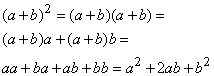Most Operations Are Not Distributive.

 What is the Distributive Law? The distributive law is the nickname for the distributive law of multiplication over addition. It says: The product of a number and the sum of other numbers is the sum of the products of the number and each of the other numbers. In symbols it says:In other words, the law says: One need not add what's in parenthesis before multiplying as directed by the inclusion marks as long as one multiplies each of the addends first by the desired multiplier. The sum of these results will be equivalent to the desired expression. It's legal to multiply first if you multiply each piece first and then add them. Why Would Anyone Want to Do This? It is more work to complete two multiplications and one addition than it is to complete one addition and one multiplication. Why would anyone want to do the extra work? Because sometime there's no other way to complete the computation. Consider these examples. LEFT EXPRESSION equals RIGHT EXPRESSION Example 1:Either expression may be completed first. Example 2:Addition in the left expression can not be completed because the terms are not like terms. The right expression is the only way to complete the desired computation. Example 3:Example 4:Example 5:Because division by c is multication by 1/c. Other Operations Do Not Distribute Because this works so nicely, one might believe this distribution of the first number to each of the other numbers works for other operations as well. Life's not that easy. Consider a few more operations where it may look as if a distributive law exists but where it does not. LEFT EXPRESSION does not equal RIGHT EXPRESSIONThe distributive law is the distributive law of multiplication over addition. Other varieties do not exist! Multiplication and division are the oly operations which distribute over addition or subtraction.

This is a page from the dictionary MATH SPOKEN HERE!, published in 1995 by MATHEMATICAL CONCEPTS, inc., ISBN: 0-9623593-5-1.   You are hereby granted permission to make ONE printed copy of this page and its picture(s) for your PERSONAL and not-for-profit use.